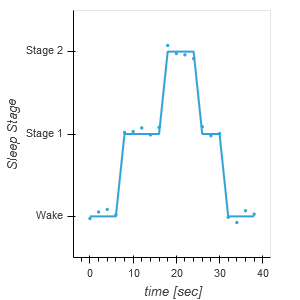# Jitter for y-axis

Hello,

I am trying to overlay a scatter plot onto a curve. The y-axis of my data is categorical and my x-axis is time. I want to jitter my scatter plot in the y-axis but it does not seem to let me. I tried inverting my axes for the scatter plot, and when I plot the scatter plot by itself it looks the way I want it, but once I try to overlay the curve plot, things get weird with the axes. Bellow is an example of the data I am working with. If you have any advice or suggestions I would much appreciate it. Thank you!

``````import pandas as pd
import hvplot.pandas
import holoviews as hv
import panel as pn
hv.extension('bokeh')

df = pd.DataFrame(columns=['time [sec]','Sleep Stage'],index=range(0,20))
df['time [sec]']  = 2*df.index
df['Sleep Stage'] = ['Wake','Wake','Wake','Wake','Stage 1','Stage 1','Stage 1','Stage 1','Stage 1','Stage 2','Stage 2','Stage 2','Stage 2','Stage 1','Stage 1','Stage 1','Wake','Wake','Wake','Wake']
scatter  = hv.Scatter(df,vdims=['time [sec]'],kdims=['Sleep Stage']).opts(jitter=0.2,invert_axes=True)
curve = hv.Curve(df,'time [sec]','Sleep Stage')

scatter*curve``````

One of the reasons I really do not like categorical axes…
You can use numerical axes and specify the axis tick labels instead.
My HoloviewsPlayground on github is getting a bit outdated, but here is an example from StatisticalPlots.ipynb:

``````import seaborn as sns
iris['species'] = iris['species'].astype('category')

def strip_plot(df,x,y,jitter=.2):
ticks = [(i,v) for i,v in enumerate(df[x].cat.categories)]
return hv.Points((np.array([df[x].cat.codes, df[y], df[x].cat.codes.astype(float)]).T), kdims=[x,y],vdims=['color'])\
.opts(xticks=ticks, color_index=x)

strip_plot( iris, 'species', 'sepal_length')\
.opts( hv.opts.Points(width=500, jitter=.4, tools=['hover'], size=5, color=hv.Cycle(['green','blue','red'])))\
.redim.range(species=(-.5,2.5),sepal_length=(4,8.5))
``````

Something weird about colors though. If anybody sees the problem, I’d love to know!

Hi, maybe I don’t understand what you want to do, but if you specify the same kdims and vdim for both your curve and your scatter you should be able to make the overlay.

``````import pandas as pd
import hvplot.pandas
import holoviews as hv
import panel as pn
hv.extension('bokeh')

df = pd.DataFrame(columns=['time [sec]','Sleep Stage'],index=range(0,20))
df['time [sec]']  = 2*df.index
df['Sleep Stage'] = ['Wake','Wake','Wake','Wake','Stage 1','Stage 1','Stage 1','Stage 1','Stage 1','Stage 2','Stage 2','Stage 2','Stage 2','Stage 1','Stage 1','Stage 1','Wake','Wake','Wake','Wake']
scatter  = hv.Scatter(df,vdims=['time [sec]'],kdims=['Sleep Stage']).opts(jitter=0.2,invert_axes=True)
curve = hv.Curve(df,vdims=['time [sec]'],kdims=['Sleep Stage'])

scatter*curve
``````Thank you! That worked.